Courses

# Introduction to Electrical & Electronics Measurements

## 10 Questions MCQ Test GATE Electrical Engineering (EE) 2022 Mock Test Series | Introduction to Electrical & Electronics Measurements

Description
This mock test of Introduction to Electrical & Electronics Measurements for Electrical Engineering (EE) helps you for every Electrical Engineering (EE) entrance exam. This contains 10 Multiple Choice Questions for Electrical Engineering (EE) Introduction to Electrical & Electronics Measurements (mcq) to study with solutions a complete question bank. The solved questions answers in this Introduction to Electrical & Electronics Measurements quiz give you a good mix of easy questions and tough questions. Electrical Engineering (EE) students definitely take this Introduction to Electrical & Electronics Measurements exercise for a better result in the exam. You can find other Introduction to Electrical & Electronics Measurements extra questions, long questions & short questions for Electrical Engineering (EE) on EduRev as well by searching above.
QUESTION: 1

### in present day measurement systems

Solution:

Measurement by direct methods are not always possible, feasible and practicable. In most of the cases direct method of measurements are inaccurate because they involve human factors. Moreover, they are less sensitive. Due to these reasons use of direct method of measurement is limited while indirect methods are commonly used

QUESTION: 2

Solution:
QUESTION: 3

### A thermometer is calibrated 150°C to 200°C. The accuracy is specified within ±0.25 percent of instrument span. The maximum static error is

Solution: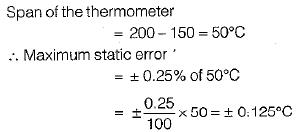QUESTION: 4

A set of independent current measurements were recorded as 10.03, 10.10, 10.08 and 10.11 A, The average range of error is

Solution:

Average value of current is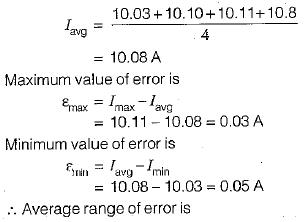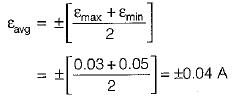QUESTION: 5

Two resistors R1, and R2 are connected in series with R1 = 28.7 Ω and R2 = 3.624 Ω. The total resistance to the appropriate number of significant figures can be written as

Solution: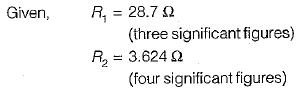For series connection,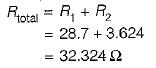Since one of the resistance (i.e. R1) is accurate only to three significant figures, therefore the result of R total has to be reduced to three significant figures. Hence, total resistance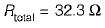QUESTION: 6

A Wheatstone bridge requires a change of 8 a in the unknown arm of the bridge to produce a change in deflection of 3 mm of the galvanometer. The deflection factor (scale factor) is

Solution: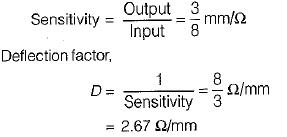QUESTION: 7

A moving coil voltmeter has a uniform scale with 100 divisions, the full scale reading is 200 V and 1/10 of a scale division can be estimated with a fair degree of certainty.The resolution of the instrument in volt is

Solution: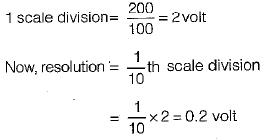QUESTION: 8

A multimeter having a sensitivity of 2 kΩ/V is used for the measurement of voltage across a circuit having an output resistance of 10 kΩ. The open circuit voltage of the circuit is 6 volts. The percentage error in multimeter reading when it is set to 10 volt is given by

Solution:

The circuit diagram for the measurement of voltage using multimeter is shown below: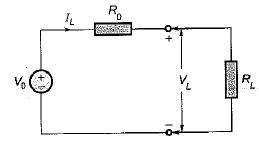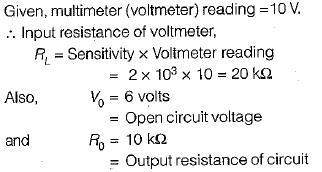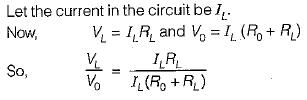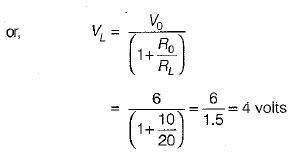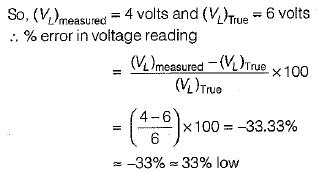QUESTION: 9

A 50 V range voltmeter is connected across the terminals X and Y of the circuit shown in figure below. The voltage across the terminals are measured both under open circuit and loaded conditions.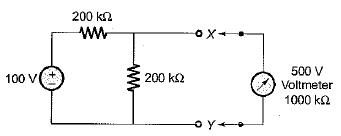The accuracy in the measurement of voltage across the terminals X and Y in percent is

Solution:

The given circuit can be reduced to its Thevenin’s equivalent circuit as shown below.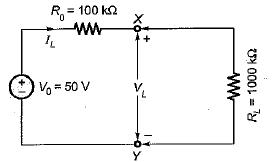True value of voltage across terminals X-Y is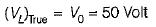Measured value of voltage across terminals X-Y is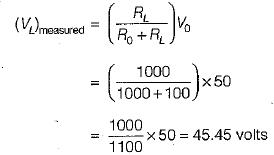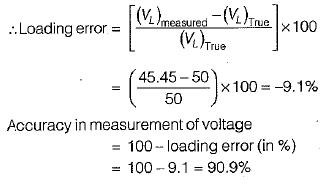QUESTION: 10

The number of significant figures in the two resistors having resistances 4 x 106 kΩ and 0.345 kΩ are respectively

Solution: